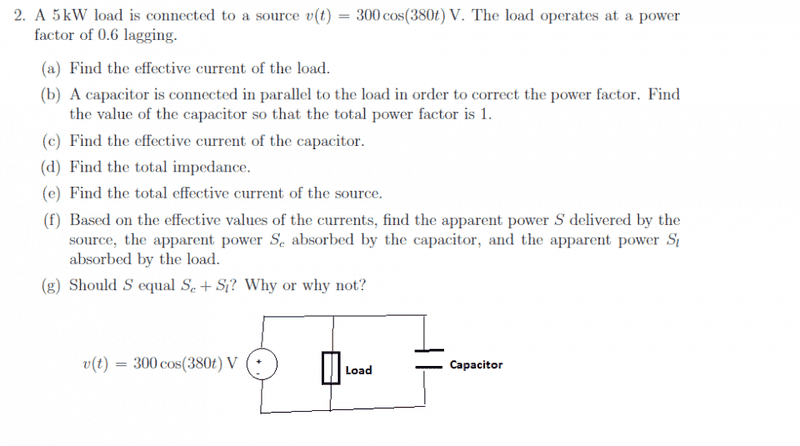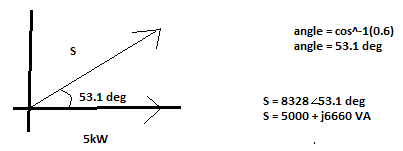# AC Power and Effective Currents

• YellowBelliedMarmot
Ieff = j*22.2058 A In summary, the effective current of the capacitor is 22.2058 A rms.f

## Homework StatementI added a circuit that I drew in microsoft paint to add a visual. Sorry for its ugliness! ;D

## Homework Equations

V = I*Z
Ieff = I / sqrt(2)
Qreactive = V^2 / Z
Pavg = (power factor) * (Veff) * (Ieff)
Sapparent = ((Ieff)*(Veff))/2

## The Attempt at a Solution

Since this is quite a long problem, I'll detail my thought process up until I get stuck. On the left, I have my work, and then I explain what I am doing on the right.

a) To find the effective current over the load:

P = (1/2)*v*i*(pf) Solve for i... (pf = power factor)
i = 2P/(v*pf) Then substitute in values...
i = (2*5000)/(300*0.6)
i =55.56 Now I have to divide the current by the square root of 2 to get the effective power
i = 55.56/sqrt(2)
i = 39.28 A rms This is the answer I get for a) and I have confirmed that it is correct.

b) Now I have to correct the power factor from 0.6 to 1 by adding a capacitor in parallel with the load.PF = 1
theta = cos^-1(1) Finding the angle when the PF is 1...
theta = 0 degrees

Qn = tan(0deg) Using the new power factor, I'm finding the new reactive power
Qn = 0

Qc = -(Ql - Qn) We can find the reactive power of the capacitor by subtracting Ql and Qnew
Qc = -(6660-0) Substituting in values...
Qc = -6660 VAR This is the reactive power of the capacitor

Q = V^2 / Z Now we use the reactive power of the cap to find its impedance...
Z = V^2 / Q Solve for Z
Z = (300^2 / -6660) Substitute
Z = -j13.51 This is the impedance of the cap

Z = -j/wc Now we have to find the capacitance...
C = -j/wZ Solve for C
C = -j / (380 * -j13.51) Put the equation into a calculator...
C = 194.8 uF I have no way of confirming if this is right, but I'm pretty confident that it is!

c) Ok... this is where things start to get a bit shaky...
So in order to find the effective current of the capacitor...

V = I * Z
I = V / Z Solve for I...
I = (300) / (-j13.51) Substitute...
I = j 22.2058 Then I have to multiply this by 1/sqrt(2) to get the effective current...
I = j 22.2058 / sqrt(2)
Ieff = 15.7 A This is the answer I get; however, I know it is wrong. It should be twice that...

Ieff = 31.4 A This is what the answer is supposed to be. I am unsure why I get half it.

d) I feel as though the snowball is growing bigger and bigger as it rolls down the hill... Or in other words, I believe a small mistake that I've made is making all the following questions incorrect.
In order to find the impedance of the load...

V = I * Z
Z = V / I Solve for Z
Z = (300) / (39.28) Substitute... (But I don't know which value to use for I... so I used Ieff)
Z = 7.64 ohms This is the impedance of the load which is in parallel with the capacitor...
Ztotal = (7.64) || (-j13.51) Finding the total impedance...
Ztotal = 5.79 - j3.27 Pretty sure this is wrong...

e) So now I need to find the effective current of the source...

V = I * Z
I = V / Z Solve for Z
I = (300) / (5.79 - j3.27) Substitute...
I = 39.28 + J22.19 Now I have to multiply this by 1/sqrt(2) to get the effective power...
I = 27.78 + j15.69
I = 27.78 A rms I know this is wrong. The answer should be 23.57 A rms.

f) Now I need to find the apparent power, S, for each the load, capacitor, and the source...

S = (Ieff * Veff) / 2

Scap = (300*31.4)/2
Scap = 5.71 kVA

Ssource = (300*23.57)/2
Ssource = 3.535 kVA I know this value is wrong since it is supposed to be 5kVA. And because this is wrong, I suspect that the others are wrong as well.

g) And the last one... It's more of an explanation, so I'll take a whack at it...
Ssource should not equal Scap + Sload because capcaitors only absorb reactive power (Q) and the Ssource is not a complex number. However, I'm conflicted on this reasoning because isn't it also the case that the power delivered should be equal to the power absorbed?

OK... So it is a pretty long problem and I appreciate it if you have stuck through the whole thing. I don't know exactly what is wrong, but I have suspicions:

1. I am unsure as to why the answer I got is exactly one half of the actual answer... perhaps I dropping a 2 somewhere? I know there are a whole bunch of new equations that get thrown around in AC Power, so I may have gotten a formula wrong.

2. For part c), I really don't think I am supposed to substitute the I effective in for I, but I don't know what I would be.

3. And then everything from part d) and on I think just stems from the previous wrong answers.

Again, thank you to all those who have been helping me through circuits! It's almost over! (^_^)

C = 194.8 uF I have no way of confirming if this is right, but I'm pretty confident that it is!
The capacitance value is incorrect.
Z = (300^2 / -6660)
You have taken the peak voltage in this formula. It should be RMS. This is where you dropped a factor of 2.

Last edited:
•YellowBelliedMarmot
The capacitance value is incorrect.

You have taken the peak voltage in this formula. It should be RMS. This is where you dropped a factor of 2.

I fixed the voltage; changing it from peak to rms...

Vpeak = 300 v

Vrms = 300 * (1/sqrt(2))
Vrms = 212.13 v

Then I used this number to solve for the impedance of the capacitor...

Q = v^2 / Z Solve for Z...
Z = v^2 / Q Substitute...
Z = (212.13^2)/-6660 Plug into calc...
Z = -j6.75 ohms Fixed impedance

Then I use the impedance to find the capacitance...

Z = -j / (wC) Solve for C...
C = -j / (wZ) Substitute...
C = -j / (380 * -j6.75) The "-j"s cancel out...
C = 1/(380 * 6.75)
C = 390 uF Fixed Capacitance...

Then I use this value to get the effective current across the capacitor...

I = V/Z Substitute...
I = (212.13) / -j6.75
I = 31.43 amps at an angle of 90 deg
Ieff = 31.43 A rms Fixed effective current

Now I am running into some difficulty again as I try to find the total impedance...

Zload = V / I Substitute the rms voltage and current (Vrms = 212.13v and Irms = 39.28A)...
Zload = (212.13) / 39.28 calculator...
The load is in parallel with the capacitor, so I'll do the product over the sum...
Ztotal = ((5.4)*(-j6.75))/((5.4)+(-j6.75)) Calculator...
Ztotal = 3.29 - j2.63 Rectangular Form...
Ztotal = 4.22 at an angle of -38.7 deg

I believe; however, that this impedance is incorrect since the total effective current is incorrect...

I = V /Z Substitute the rms voltage and impedance in (Vrms = 212.13 and Ztotal = 3.29 -j2.63)...
I = 212.13 / (3.29 -j2.63)
I = 39.3 + j31.4 Rectangular form...
I = 50.36 at an angle of 38.6 deg Polar form...

However, I have the confirmed total effective current and it is I = 23.57 A rms...
If I had to guess what I did wrong, like you said earlier, I think I am confusing when I should use rms values and peak values. Either that or I calculated the impedance incorrectly. Oh and I fixed the last couple parts... I tried to divide the already rms value of the current by another square root... I guess I tried to invent a new sort of double rms current out of my ignorance! Thank you for your help with this! I've been having an incredibly difficult time with circuits... ;D

Ztotal = 3.29 - j2.63 Rectangular Form...
Ztotal = 4.22 at an angle of -38.7 deg
After addition of the capacitor in the circuit, power factor becomes 1. Hence, your Ztotal should be purely resistive i.e. without any imaginary part.

•YellowBelliedMarmot
Ztotal = ((5.4)*(-j6.75))/((5.4)+(-j6.75))
You are treating the 5.4 ohm impedance as a resistance only. You should write it in the complex form as R+jXL. After simplification, you'll have a purely resistive Ztotal.

•YellowBelliedMarmot
You are treating the 5.4 ohm impedance as a resistance only. You should write it in the complex form as R+jXL. After simplification, you'll have a purely resistive Ztotal.

I think I understand what you're trying to get at, but I just don't exactly know how to get there.

Here's where I'm not exactly making the connection...

So the effective voltage across the load is 212.13 v and the effective current is 39.28 A. By dividing the voltage by the current, I get the 5.4 ohm impedance which you mentioned in purely resistive (since it is doesn't have any imaginary part). Up until this point, I understand and I know that I must write it in its complex form; however, I am confused as to how to get it. Since the effective current and voltage are both real numbers, how would I get the complex number from them? Or is it that I am using the wrong number for the current and or voltage which is producing an incorrect impedance?

As a sort of refresher, I'll add how I got each of the effective current/voltage...

I = 2P/(v*(PF))
I = (2*5000)/(300*0.6)
Ieff = 55.5 * (1/sqrt(2))
Ieff = 39.28 A rms

Veff = 300 * (1/sqrt(2))
Veff = 212.13

I get the 5.4 ohm impedance which you mentioned in purely resistive (since it is doesn't have any imaginary part).
This is your load impedance, which is inductive in nature since it is at 0.6 pf lagging (as mentioned in the problem). You should write this load impedance of 5.4 ohm as R+jX, where R=ZcosΦ and X=ZsinΦ. Then you'll have that R+jX in parallel with -j6.75 ohm for Ztotal. Your Ztotal must be purely resistive since the power factor became unity after addition of the capacitor.

•YellowBelliedMarmot
This is your load impedance, which is inductive in nature since it is at 0.6 pf lagging (as mentioned in the problem). You should write this load impedance of 5.4 ohm as R+jX, where R=ZcosΦ and X=ZsinΦ. Then you'll have that R+jX in parallel with -j6.75 ohm for Ztotal. Your Ztotal must be purely resistive since the power factor became unity after addition of the capacitor.

Ok! When I do that I get for the R + jX...

Z = 3.24 + j4.32 which is in parallel with the -j6.75
Ztotal = 9

Then to find the total effective current...

I = V / Z
I = 212.13/9
I = 23.57 A rms which is the correct answer! :)

Thank you for your help! I have been stuck on this problem for what seems like ages! I'm in an 8 week online course and I have had no prior experience with circuits. My time so far has been incredibly stressful having to teach myself, and I really do appreciate your help!

•cnh1995
I think I am confusing when I should use rms values and peak values.
When dealing with rectangular and polar forms of voltage and current, always use peak values. When writing KCL and KVL, use rms quantities for voltage and current.

•YellowBelliedMarmot